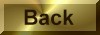Practice Problem 9

Explain why 0.60 grams of acetic acid dissolve in 200 grams of benzene to form a solution that lowers the freezing point of benzene to 5.40oC.

Because pure benzene freezes at 5.53oC, the freezing point depression in this experi- ment is -0.13oC.TFP = 5.40oC - 5.53oC = -0.13oC

Once again, we can start with the relationship between the freezing point depression and the molality of the solution.TFP = -kfm

We then use the molal freezing point depression constant for benzene to calculate the molality of the solution.

 m =TFP = 0.13oC = 0.025 m kf 5.12oC/m

Multiplying the molality of the solution by the amount of solvent used to make the solution gives the number of moles of solute particles in the solution.

 0.025 mol solute x 0.200 kg benzene = 0.0050 mol solute 1 kg benzene

Since the solution contains 0.60 grams of acetic acid, the molecular weight of acetic acid in this experiment is 120 grams per mole.

 0.60 g solute = 120 g/mol 0.0050 mol solute

The molecular weight of acetic acid in benzene is twice as large as what is expected from the molecular formula, CH3CO2H. This can be explained by assuming that acetic acid molecules associate in benzene to form dimers, which are held together by hydrogen bonds, as shown in the figure below.